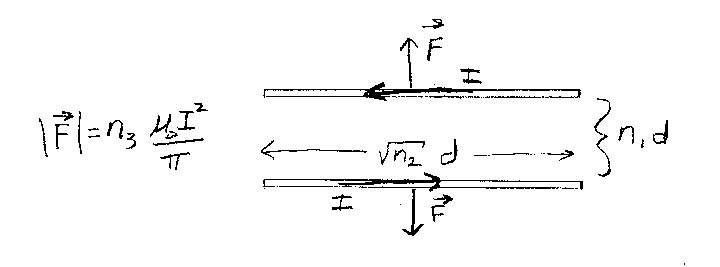Problem C16: In the figure below there are two wires, each of finite length n2d. Each wire carries a current I. The wires are parallel to each other and are separated by a distance n1d. What is the net magnetic force that one finite wire exerts on the other finite wire? That is, if the net magnetic force that one finite wire feels due to the other is F = n30 I2)/π , what is n3? Note that n1, n2, and n3 are unitless.n1 = n2 = Input n3:
If you are currently in my class, you can record your grade by entering your name and student ID number (without the leading zeros) below and clicking on "record grade".
 First Name = Last Name = ID = Problem: